Pakdil, N. B., and Balaban, S. (2022). "Assessment of non-pressurized electro dewatering of waste activated sludge with graphite electrodes," BioResources 17(2), 3355-3377.

#### Abstract

Changes of physical and chemical characteristics of sludge were investigated with respect to the pH, process time, and applied voltage by means of the electric field treatments. A model reactor with the desired speed was used to generate an electric field to separate water from the sludge without applying pressure. All electrodes mounted on the reactor were produced from graphite. Two different distances between anode and cathode (2.3 cm and 4.3 cm) were used to examine the effects on dewatering capacity of sludge. Differentiations of dependent variables inferred from the experimental processes were also surveyed by Box-Behnken experimental design. The water in the sludge was separated effectively when the samples were exposed to an electric field. The total solid increased from 1.07% to 6.60% at pH 6.5 when sludge was exposed to 25 V for 60 min. Furthermore, the optimum distance between electrodes was 2.3 cm for dewatering of sludge samples, where the capillary suction time of influent sludge was observed to decrease by approximately 85.5% at raw sludge pH. Similarly, the viscosity parameters decreased by approximately 99%. There was an increase in the soluble chemical oxygen demand, PO4-P, and NH4-N concentrations of water discharged from the model reactor.

Assessment of Non-Pressurized Electro Dewatering of Waste Activated Sludge with Graphite Electrodes

Nazlı Baldan Pakdil* and Serap Balaban

Changes of physical and chemical characteristics of sludge were investigated with respect to the pH, process time, and applied voltage by means of the electric field treatments. A model reactor with the desired speed was used to generate an electric field to separate water from the sludge without applying pressure. All electrodes mounted on the reactor were produced from graphite. Two different distances between anode and cathode (2.3 cm and 4.3 cm) were used to examine the effects on dewatering capacity of sludge. Differentiations of dependent variables inferred from the experimental processes were also surveyed by Box-Behnken experimental design. The water in the sludge was separated effectively when the samples were exposed to an electric field. The total solid increased from 1.07% to 6.60% at pH 6.5 when sludge was exposed to 25 V for 60 min. Furthermore, the optimum distance between electrodes was 2.3 cm for dewatering of sludge samples, where the capillary suction time of influent sludge was observed to decrease by approximately 85.5% at raw sludge pH. Similarly, the viscosity parameters decreased by approximately 99%. There was an increase in the soluble chemical oxygen demand, PO4-P, and NH4-N concentrations of water discharged from the model reactor.

DOI: 10.15376/biores.17.2.3355-3377

Keywords: Electro-dewatering; Graphite; Model reactor; Response surface method; Sludge

Contact information: Department of Environmental Engineering, Faculty of Engineering, Bolu Abant Izzet Baysal University, Bolu, Turkey; *Corresponding author: nazlipakdil@gmail.com

INTRODUCTION

The disposal of sludge is both difficult and expensive, requiring more than 50% of the operating budget of a wastewater treatment plant (WWTP) (Spinosa and Vesilind 2001). It has been reported that 30 to 50% of annual operating costs are related to sludge dewatering (Sorensen 1996; Olivier et al. 2014). The reduction of sludge volume by solid-water separation is very important in terms of reducing its disposal cost (Cao et al. 2019). The water content of sludge is usually reduced by mechanical processes (e.g., centrifuges, belt filter presses, or filter presses) due to their advantages such as simplicity, ubiquity, and relatively low costs. Although the solid material of activated sludge (~35%) appears to be the highest value, the moisture content (MC) of activated sludge is still very high (Loginov et al. 2013; Olivier et al. 2014; Sha et al. 2019).

The target amount of water to be removed from the sludge and water distribution within the material are important parameters affecting the efficiency of dewatering method. Four different types of water are present in the sludge water distribution model (Vesilind 1994). These are (I) free water, (II) interstitial water, (III) vicinal water, and (IV) bound water in the sludge. Mechanical dewatering methods are effective on free water and small amounts of interstitial water (Zhou et al. 2001). Therefore, the increment in the dewatering efficiency is crucial for reducing the cost of sludge disposal. The most efficient method selected is controlled by the physical components of sludge, and the optimal sludge moisture content reduces the energy and minimizes the environmental cost.

Electro-dewatering (EDW) is performed by exposure to an electric field, which induces an electrokinetic transport of the liquid phase and thus enhances the efficiency of dewatering (Mahmoud et al. 2010; Ammami et al. 2020). The EDW comprises the stages of a filter cake formation and consolidation, assisted by four electrical field induced-phenomena: electrophoresis, electroosmosis, electrolysis, and ohmic heating (Weber and Stahl 2003; Mahmoud et al. 2010; Loginov et al. 2013). Electro-osmosis depends on the dispersion medium in the direction of the oppositely changed electrode. Electro-osmosis occurs when the suspended fluid flows in one direction. Electro-migration (also known as ion migration) occurs when the charged ions in the liquid phase migrate towards the electrodes of opposite polarity (Glendinning et al. 2007; Mahmoud et al. 2010).

The properties of sludge, treatment duration, and electrode selection are important parameters affecting the EDW process. The sludge properties vary based on the duration of sludge formation and type of wastewater. The duration ranges from a few min to a few hours, which plays a major role on the electro-dewatering process. Consequently, the duration should be within a certain limit. If electro-dewatering is too short, the process will not be completed with the necessary efficiency (Smollen and Kafaar 1994; Snyman et al. 2000). If the process is too long, the efficiency will decrease due to the increase in energy consumption (Gingerich et al. 1999; Barton et al. 2007).

The selection of anode and cathode plays an important role in reactions during the process. Oxidation, corrosion, and precipitation reactions take place on the electrode surface under the external electric field. The electrode reactions depend on electrode material and ions present in the electrolyte (Tuan et al. 2008). Many different materials can be used as the electrode materials, for example, materials coated with mixed metal oxides, stainless steel, and titanium for the anode and stainless steel or materials coated with mixed metal oxides for the cathode (Qian et al. 2015; Gronchi et al. 2017; Zhang et al. 2017; Yang et al. 2018). Graphite is the preferred electrode material in many EDW processes due to its superior features including low-cost material and effective behavior (Yuan and Weng 2003; Chu et al. 2005; Yang et al. 2005; Reddy et al. 2006; Mu’azu and Essa 2020). In addition, it is effective as anode electrode in the electrochemical oxidation of paracetamol in water (Periyasamy and Muthuchamy 2018). Furthermore, the EDW performance of sludge is increased by adding graphite particulates (Cao et al. 2019).

That the application of electric field to the sludge has an effect on the soluble chemical oxygen demand (SCOD) of the sludge has been observed. It was determined that the organic matter was decomposed and solubilized depending on the input power and the electrolysis time; thus, the SCOD concentration in sludge increased (Song et al. 2010). The COD and TOC concentrations in removed water by applying electric field were especially higher at the anode (Tuan et al. 2008). Similarly, it was found that phosphorus and ammonium concentration in removed water increased (Song et al. 2010).

EDW studies focus mostly on systems formed by the pressurized electric current (Gronchi et al. 2017; Zhang et al. 2020; Sha et al. 2021a; Liu et al. 2021), while non-pressured EDW systems rarely have been used (Tuan et al. 2008; Qian et al. 2015). In this study, a non-pressured EDW system was developed to examine the effect of external electric field on dewatering efficiency by removing the pressure factor. The other objectives were to determine the effect of a tubular graphite electrode on EDW process, to optimize properties of sludge dewaterability, and to evaluate the changes of physical and chemical characteristics in the sludge vs. pH, process time, and applied voltage.

EXPERIMENTAL

Sludge Samples and Characterization

Waste activated sludge samples were collected from the sludge return line of the secondary clarifier of a municipal WWTP in Bolu (Turkey). The plant treats 39,000 m3/d of wastewater with the conventional activated process. Samples was stored for 24 h at 4 °C. The capillary suction time (CST), indicating how quickly the sludge releases its intrinsic water, was measured to evaluate the sludge dewatering behavior (Yuan et al. 2010). The CST of sludge samples was determined using a CST Instrument (Triton 304M, Essex, UK) with Whatman No 17-filter paper. The specific resistance to filtration (SRF) test was performed by means of a 9-cm standard Büchner funnel with Whatman No 2-filter paper. The vacuum suction was adjusted to 35 kPa. The values of SRF were inferred from the slope of a linear plot of volume versus time/volume (Lo et al. 2001). Total solids (TS) and volatile suspended solids (VSS) content of sludge samples were determined by drying overnight at 100 ± 3 °C (2540 D) and by ignition at 550 °C for 20 min (2540 E), respectively. The samples were centrifuged at 4500 rpm for 15 min (Nüve NF200) and then filtered through a 0.45 µm filter. The samples were prepared for the SCOD, PO4-P, and NH4-N analyses using a spectrophotometer -S. Pharo 100 (Merck, St. Louis, MO, USA) as described in the APHA (2005) standard. The viscosity was detected with a Brookfield DV2TLV viscometer (Preshaw, UK). The pH was measured using a pH meter (Hanna Instruments HI 2211, USA). After determining the pH value of the raw sludge, the initial pH of the raw sludge to add the model reactor was adjusted to be pH 5 and 8 with the aid of NaOH and HNO3 solutions according to the statistical experimental design. The sludge characteristics are presented in Table 1.

Table 1. Activated Sludge Characteristics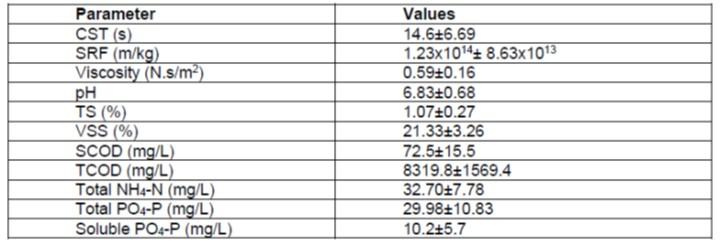Non- Pressurized EDW Model Reactor Configuration

Both the horizontal and vertical electric fields were generated in laboratory scale EDW equipment. According to the position of electrodes, the sludge volume can be reduced by changing the electric field. There have been limited studies on the form and structure of EDW equipment (Sun et al. 2021). In this study, fixed graphite electrodes were used for the electro-dewatering process. The anode and cathode remained stable as the model reactor was rotated around its own axis. The sludge between the two electrodes was moved with the model reactor in the same direction of rotation. The purpose of rotation was to accelerate the separation of water and solid particles. In other words, the centrifugal force made the solid particles accelerate to the cathode electrode of model reactor, and the water was directed toward the cathode electrode. The aim was to send the water to the cathode electrode and separate water and solid particles by rotating the model reactor at 80 rpm. The effluent water removed during the process was taken out of the non- pressurized EDW model reactor, and its content was analyzed. The dewatered sludge remaining between the electrodes was removed by scraping it off at the end of the processing time. (Fig. 1).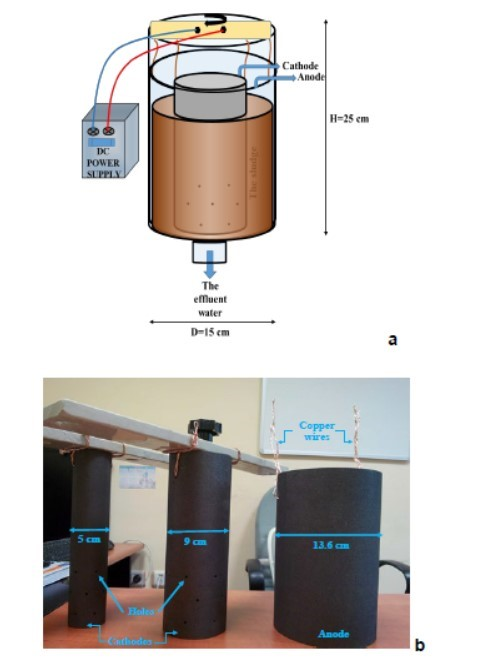Fig. 1. Non- Pressurized EDW Model Reactor (a) and the graphite electrodes (b)

The model reactor, made from Plexiglas, was 15 cm in diameter and 25 cm in height. The outer diameter of electrode used as an anode (except for wall thickness) was 13.6 cm, while the outer diameters of electrodes used as a cathode were 5 and 9 cm, respectively, to examine the effect of distance between the electrodes. Holes were opened on the cathode electrode to allow the passage of effluent water. A limited number of holes in the electrodes were drilled to prevent weakening the graphite material and so that there was no obstacle to the passage of water. A total of 14 holes with a diameter of 3 mm were drilled on each cathode. Also, holes were drilled in the upper part of electrodes to allow the copper wires to provide electrical conduction. The model reactor system was designed to open to the atmosphere by taking into the account release the hydrogen gas (Eq. 1 and Eq. 2) that was generated by the cathode (Sha et al. 2021b).

The reaction at the anode was,

2H2O- 4e →O2↑ + 4H+ E0 = 1.229 V (1)

whereas the reaction at the cathode was:

2H2O+2e →H2↑+ 2OH E0 = – 0.828 V (2)

Statistical Design

A Box-Behnken experimental design was used to define the effects of pH, process time, and voltage on TS, CST, viscosity, SCOD, PO4-P, and NH4-N concentrations and to determine optimum values of independent variables (pH, process time, and voltage). Box and Behnken proposed three-level experimental designs (Eq. 3) for fitting response surfaces (Box and Behnken 1960; Montgomery 2013),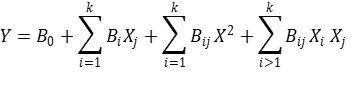(3)

where Y is the predicted response, B0 is a constant, and Bi and Bij are the cross-product and quadratic coefficient, respectively. The response function coefficients were analyzed using MINITAB 17.1 Statistical Software Program (State College, PA, USA).

The Box-Behnken experimental design was chosen to evaluate the effects of multiple variables on every test run. In addition, the Box-Behnken design offers the advantage of requiring fewer test runs for three factors. The coded and actual values of explanatory variables of (I) pH (X1), (II) process time (X2), (III) voltage (X3), and the responses used in the experimental design are given in Table 2.

Table 2. The Coded and Actual Values of the Variables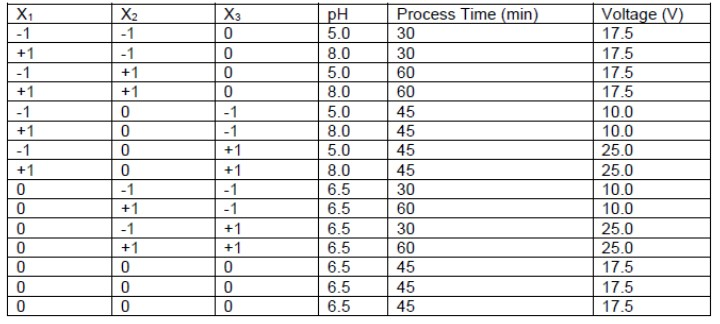RESULTS AND DISCUSSION

The constant, cross-product, and quadratic coefficient (B0, Bi, and Bij) were calculated, and the predicted response equation was determined using statistical software. The predicted response equations of TSE, CSTE, and ViscosityE for the electrode distance of 4.3 and 2.3 cm are given in Table 3. The R2 value can be interpreted as the proportion of variability in the data explained by the ANOVA model, and R2adj refers to the variation of ordinary R2 statistic that reflects the number of factors in the model (Montgomery 2013).

Table 3. Predicted Response Equations, R2 and R2adj for 4.3 cm and 2.3 m the Electrode Distance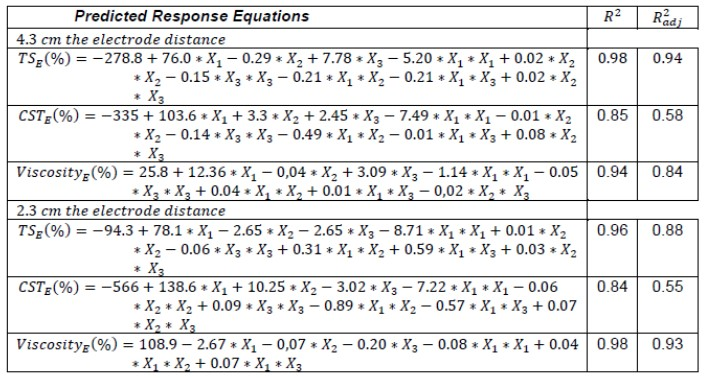Effect of the Electrode Distance on TS

The TS removal efficiency (TSE) was calculated using Eq. 4,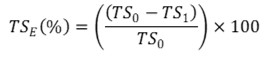(4)

where TS0 and TS1 are related to the TS of sludge before and after processing, respectively. The TSE varied with respect to the change of pH (Fig. 2). Namely, lower pH resulted in more TS removal efficiency even if the voltage duration was kept stable (approximately 40% at pH 5 and 20% pH 8 for 60 min). However, TS removal was no longer observed at or above pH 8. Moreover, the efficiency increased by 8% (noted as the optimum efficiency value) when the process duration was increased from 30 min to 60 min at the constant pH of 5. As for the variation of TSE against the applied voltage, the increment in the voltage value from 10 V to 25 V was observed to reduce considerably the TSE.

It was observed that TS removal efficiency was respectively 50% and 20% at pH 5 and 8 during 60 min processing time. The most interesting results were observed for 2.3 cm between anode and cathode (Fig. 3). An increment in the process duration caused an enhancement in the efficiency at the constant pH of 5. The TS efficiency value reached its maximum point (efficiency of 60%) as the process was performed at the applied voltage of 25 V for 60 min. In this respect, the optimization tests were conducted at 2.3 and 4.3 cm distances between the electrodes for the purpose of evaluating the TS removal efficiency. Based on the experimental data, the optimum process duration and voltage values were 60 min and 25 V for both tests. However, the optimum pH value was 6.39 for the test performed at 2.3 cm, while pH value of 5.57 was the best for the highest TS removal efficiency during the test conducted at 4.3 cm (Fig. 3).

The dewatering rate has been found to increase with the enhancement of the applied voltage, and hence the amounts of dry sludge systematically enhance based on the voltage value (Olivier et al. 2014). The dry solid (DS) percentage of sludge dewatered between two electrodes of model reactor was 6.6±1.1% for the voltage of 25 V during 60 min at pH 6.5. The dry solid percentage of sludge was higher than its original DS percentage of 1.07±0.27 (Fig. 4). Tuan et al. (2008) revealed similar results, showing that in non-pressure electro-dewatering process DS content in the sludge cake increased from 13.5% to 16.3% in the experiments using raw sludge.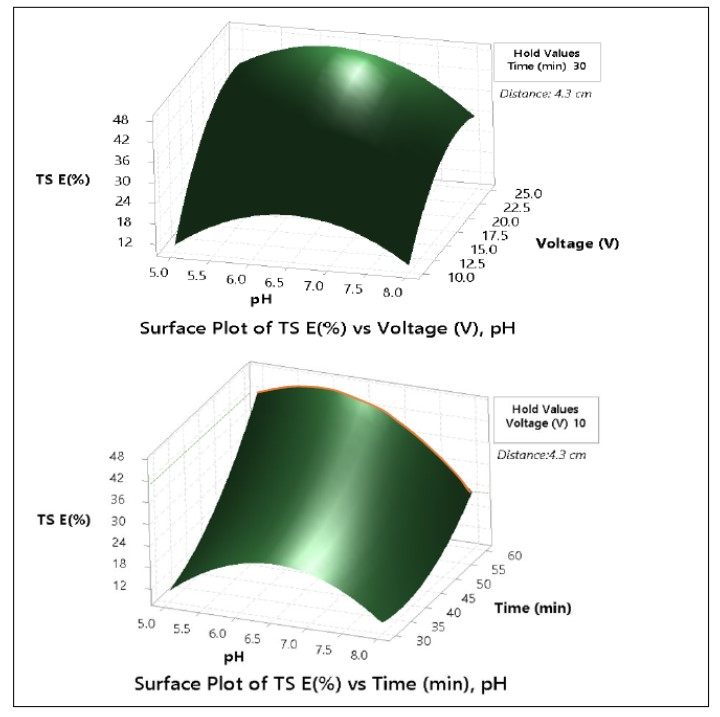Fig. 2. Surface plot of TSE (%) versus voltage, time and pH for distance 4.3 cm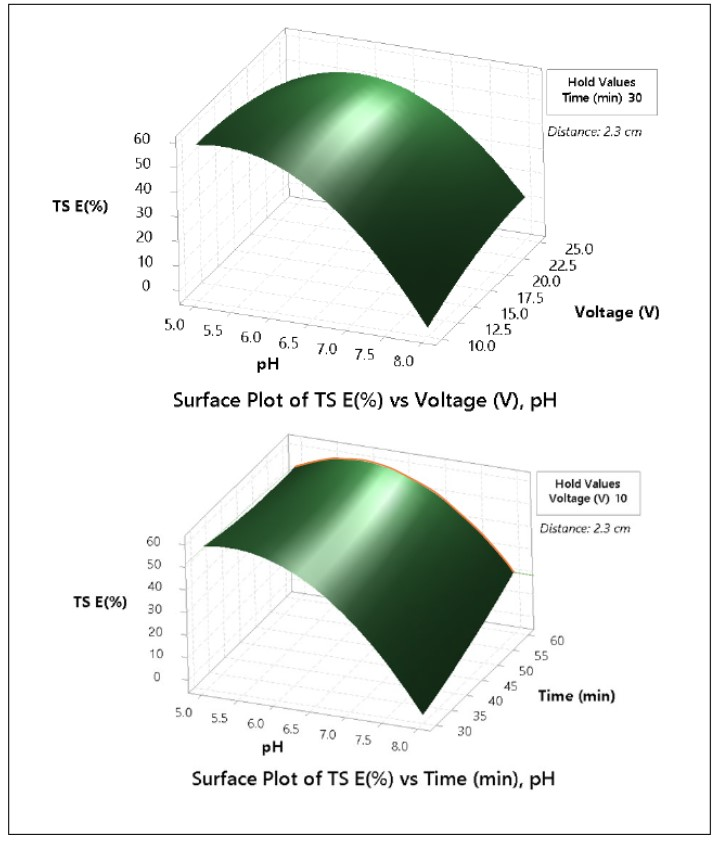Fig. 3. Surface plot of TSE (%) versus voltage, time and pH for distance 2.3 cm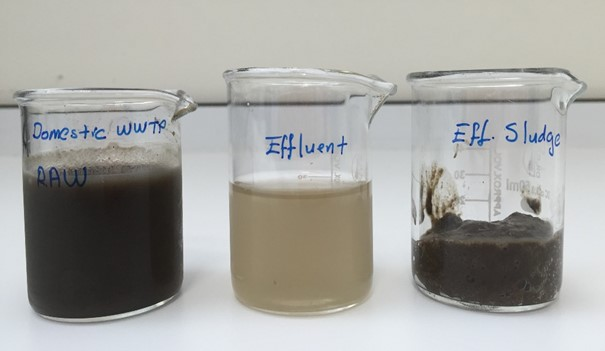Fig. 4. Influent and effluent sludge samples

Effect of the Electrode Distance on CST

CST experiment results were calculated as “CST reduction efficiency” because the experiment was performed on the sludge samples taken from the same point of the treatment plant at different times. The CST reduction efficiency (CSTE) was calculated by Eq. 5 where CST0 and CST1 are the CST of sludge before and after processing, respectively (Yuan et al. 2010).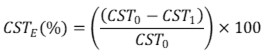(5)

In addition, CST analysis was performed at 25 ± 2 °C in consideration of the CST-temperature relationship. Experiments performed using an anode and cathode electrodes spaced at 4.3 cm showed that the pH affected the system. Namely, CSTE increased at pH 5 to 6.5 when the process duration was limited to 30 min.

As a result of the statistical optimization of maximum CSTE at pH 5 and 6.5, the experiment was performed for 60 min at 25 V, and the maximum value of CSTE was found to be 96.9% for the former pH value and 78.5% at the latter pH value for distance 4.3 cm. Considering that the pH of raw sludge was 6.83 ± 0.68, the acidification of inlet sludge led to an 18% increase in CSTE.

Therefore, the processing at pH values of raw inlet sludge did not cause a substantial difference in the efficiency of electro-dewatering as compared with CSTE values (Fig. 5). Running the system at 10 V confirmed the effect of pH. The CSTE was reduced as the pH increased to basic values; in fact, the CSTE decreased to values below zero at pH 8.

As for the change in the CSTE parameter determined at the distance between electrodes of 2.3 cm, the statistical evaluation of CSTE parameter for the inlet sludge of pH 5 and pH 6.5 showed that the highest CSTE value was obtained to be 99.7% at pH 5 and 86.3% at pH 6.5 as the process was exerted at 25 V for 60 min (Fig. 6). The CSTE increased by 13% when the inlet sludge was acidified. It should be emphasized that the effect of pH on the CSTE degraded slightly when the distance between electrodes was reduced from 4.3 cm to 2.3 cm. In addition, the shorter duration at low voltage values led to better CST reduction efficiency (Fig. 6).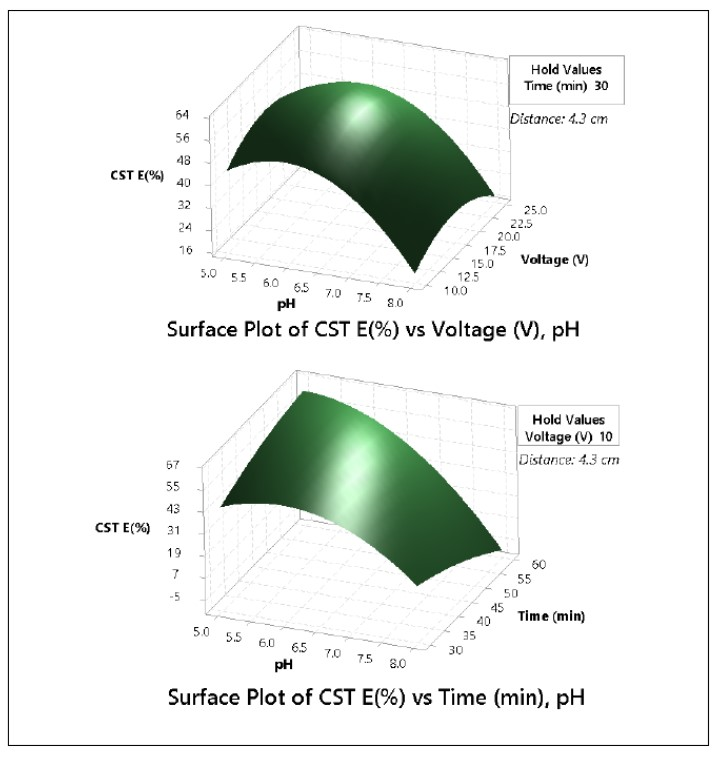Fig. 5. Surface Plot of CSTE (%) versus voltage, time and pH for distance 4.3 cm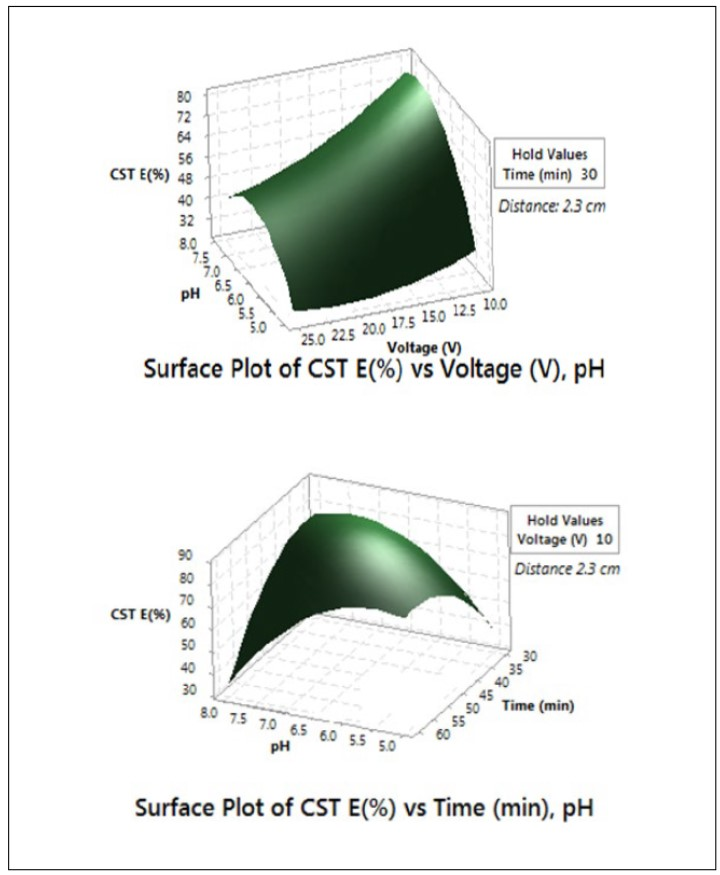Fig. 6. Surface Plot of CSTE (%) versus voltage, time and pH for distance 2.3 cm

Table 4 shows the maximum CSTE ratio calculated using the Box-Behnken design for optimization at pH 6.83. The CSTE reached 85.5% when the raw sludge was processed at low voltage (10 V) for approximately 42 min at an electrode distance of 2.3 cm. In a study conducted by Yuan (2010), the CST reduction efficiency was 18.8 ± 3.1% as a result of conditioning of sewage sludge with electrolysis at 21 V for 12 min by keeping 5 cm between the electrodes. The CSTE ratio was increased with decreased electrode distance.

Table 4. The Optimization Results for CSTE (%) Hold at pH 6.83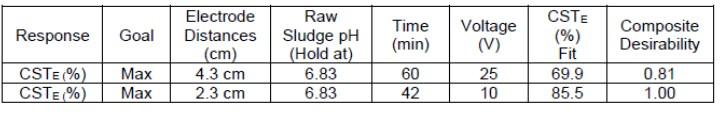Effect of the Electrode Distance on Viscosity of Effluent

The percentage of reduction in the viscosity of effluent was calculated considering the fact that the viscosity of effluent is lower than the sludge introduced into the reactor. In the present study, all the samples were analyzed at room temperature (25 ± 2 °C). The reduction of viscosity efficiency (ViscosityE) in the effluent water taken from the reactor was computed by Eq. 6 where viscosity0 and viscosity1 are related to the viscosity of sludge before and after processing, respectively.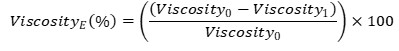(6)

As deduced from Fig. 7, the viscosity of effluent was 99% lower than the viscosity of sludge being exposed to the electric field at the constant pH of 6.5. The gradually increased voltage reduced the viscosity values at all the electrode distances, leading to the positive effects on the system performance. In other words, the water bonded with sludge separated effectively. The enhancement in the process duration along with the experiments did not lead to any significant changes in the efficiency. When the electrolysis voltage exceeds the optimum value, the dewatering might be exacerbated due to either the increase in the viscosity or the decrease in the particle size (Yuan et al. 2010). Accordingly, 25 V was judged to be the optimum voltage as a consequence of the maximum Viscosity E (%) value. In other words, the voltage value applied for 30 min is sufficient to obtain lower viscosity with the effluent. However, the electrical resistance due to the applied voltage results in ohmic heating (Joule effect). Consequently, the reduction in viscosity with the increased ohmic heating facilitates the removal of remaining water (Barton et al. 2007) and improves the dewatering capacity of sludge owing to the enhancement in Viscosity E (%) value.

Optimization of Properties of Sludge Dewaterability

The obtained responses were processed to evaluate the effects of independent variables and to find the optimum analysis conditions. The best results were obtained using 56 min of process time at pH 5.33 and 21 V of voltage for 4.3 cm of electrode distances. In addition, the optimum values of independent variables for 2.3 cm of electrode distance were 60 min of process time at pH 6.33 and 25 V of voltage (Table 5). However, the electro-dewatering improved with the reduction of distance between the electrodes when the influent sludge of pH increased. The dewaterability properties can be improved at 2.3 cm of electrode distances seriously without the change of pH of influent sludge (the raw sludge pH: 6.83). Zhan et al. (2016) concluded that the sludge with a water content of 82.1% can be dewatered to 62.2% by applying the moving-anode method with a voltage gradient of 8 V/cm and an initial electrode spacing of 1 cm.

Table 5. The Optimum Values of Variables for Maximum Values of Responses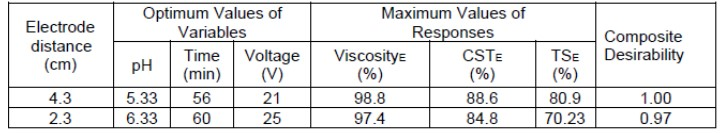Effect of the Electrode Distance on SCOD

When the initial soluble chemical oxygen demand of sludge sample was measured as 73 mg/L, the SCOD value of effluent tended to increase during the electrical current application. The effect on the system was shown based on the enhancement of concentration. Namely, the SCOD concentrations were far higher in all experiment phases than in the inlet sludge. Accordingly, Eq. 7 is used to calculate the SCOD release efficiency (SCODR) in effluent.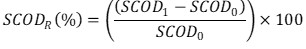(7)

The SCOD value increased by nearly 90% at every experimental measurement exposed to the electric field applications. In fact, the SCOD value reached the maximum points at both the electrode distances (2.3 cm and 4.3 cm) for the process time of 45 min as depicted in Fig. 8. In the relation, the abbreviations of SCOD0 and SCOD1 demonstrate the SCOD of sludge before and after processing, respectively. Table 5 shows the optimization results at pH 6.83 for the maximum SCOD release efficiency (SCODR).

Table 6. Predicted Response Equations, R2 and R2adj for 4.3 cm and 2.3 m the Electrode Distance